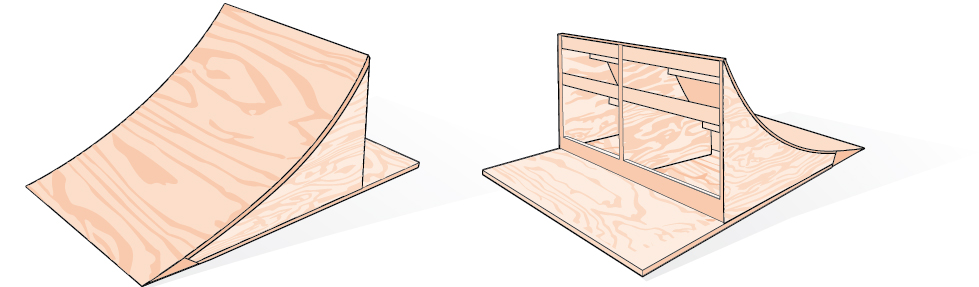# 9.3 Double-angle, half-angle, and reduction formulas

 Page 1 / 8
In this section, you will:
• Use double-angle formulas to find exact values.
• Use double-angle formulas to verify identities.
• Use reduction formulas to simplify an expression.
• Use half-angle formulas to find exact values.Bicycle ramps for advanced riders have a steeper incline than those designed for novices.

Bicycle ramps made for competition (see [link] ) must vary in height depending on the skill level of the competitors. For advanced competitors, the angle formed by the ramp and the ground should be $\text{\hspace{0.17em}}\theta \text{\hspace{0.17em}}$ such that $\text{\hspace{0.17em}}\mathrm{tan}\text{\hspace{0.17em}}\theta =\frac{5}{3}.\text{\hspace{0.17em}}$ The angle is divided in half for novices. What is the steepness of the ramp for novices? In this section, we will investigate three additional categories of identities that we can use to answer questions such as this one.

## Using double-angle formulas to find exact values

In the previous section, we used addition and subtraction formulas for trigonometric functions. Now, we take another look at those same formulas. The double-angle formulas    are a special case of the sum formulas, where $\text{\hspace{0.17em}}\alpha =\beta .\text{\hspace{0.17em}}$ Deriving the double-angle formula for sine begins with the sum formula,

$\mathrm{sin}\left(\alpha +\beta \right)=\mathrm{sin}\text{\hspace{0.17em}}\alpha \text{\hspace{0.17em}}\mathrm{cos}\text{\hspace{0.17em}}\beta +\mathrm{cos}\text{\hspace{0.17em}}\alpha \text{\hspace{0.17em}}\mathrm{sin}\text{\hspace{0.17em}}\beta$

If we let $\text{\hspace{0.17em}}\alpha =\beta =\theta ,$ then we have

$\begin{array}{ccc}\hfill \mathrm{sin}\left(\theta +\theta \right)& =& \mathrm{sin}\text{\hspace{0.17em}}\theta \text{\hspace{0.17em}}\mathrm{cos}\text{\hspace{0.17em}}\theta +\mathrm{cos}\text{\hspace{0.17em}}\theta \text{\hspace{0.17em}}\mathrm{sin}\text{\hspace{0.17em}}\theta \hfill \\ \hfill \mathrm{sin}\left(2\theta \right)& =& 2\mathrm{sin}\text{\hspace{0.17em}}\theta \text{\hspace{0.17em}}\mathrm{cos}\text{\hspace{0.17em}}\theta \hfill \end{array}$

Deriving the double-angle for cosine gives us three options. First, starting from the sum formula, $\text{\hspace{0.17em}}\mathrm{cos}\left(\alpha +\beta \right)=\mathrm{cos}\text{\hspace{0.17em}}\alpha \text{\hspace{0.17em}}\mathrm{cos}\text{\hspace{0.17em}}\beta -\mathrm{sin}\text{\hspace{0.17em}}\alpha \text{\hspace{0.17em}}\mathrm{sin}\text{\hspace{0.17em}}\beta ,$ and letting $\text{\hspace{0.17em}}\alpha =\beta =\theta ,$ we have

$\begin{array}{ccc}\hfill \mathrm{cos}\left(\theta +\theta \right)& =& \mathrm{cos}\text{\hspace{0.17em}}\theta \text{\hspace{0.17em}}\mathrm{cos}\text{\hspace{0.17em}}\theta -\mathrm{sin}\text{\hspace{0.17em}}\theta \text{\hspace{0.17em}}\mathrm{sin}\text{\hspace{0.17em}}\theta \hfill \\ \hfill \mathrm{cos}\left(2\theta \right)& =& {\mathrm{cos}}^{2}\theta -{\mathrm{sin}}^{2}\theta \hfill \end{array}$

Using the Pythagorean properties, we can expand this double-angle formula for cosine and get two more variations. The first variation is:

$\begin{array}{ccc}\hfill \mathrm{cos}\left(2\theta \right)& =& {\mathrm{cos}}^{2}\theta -{\mathrm{sin}}^{2}\theta \hfill \\ & =& \left(1-{\mathrm{sin}}^{2}\theta \right)-{\mathrm{sin}}^{2}\theta \hfill \\ & =& 1-2{\mathrm{sin}}^{2}\theta \hfill \end{array}$

The second variation is:

$\begin{array}{ccc}\hfill \mathrm{cos}\left(2\theta \right)& =& {\mathrm{cos}}^{2}\theta -{\mathrm{sin}}^{2}\theta \hfill \\ & =& {\mathrm{cos}}^{2}\theta -\left(1-{\mathrm{cos}}^{2}\theta \right)\hfill \\ & =& 2\text{\hspace{0.17em}}{\mathrm{cos}}^{2}\theta -1\hfill \end{array}$

Similarly, to derive the double-angle formula for tangent, replacing $\text{\hspace{0.17em}}\alpha =\beta =\theta \text{\hspace{0.17em}}$ in the sum formula gives

$\begin{array}{ccc}\hfill \mathrm{tan}\left(\alpha +\beta \right)& =& \frac{\mathrm{tan}\text{\hspace{0.17em}}\alpha +\mathrm{tan}\text{\hspace{0.17em}}\beta }{1-\mathrm{tan}\text{\hspace{0.17em}}\alpha \text{\hspace{0.17em}}\mathrm{tan}\text{\hspace{0.17em}}\beta }\hfill \\ \hfill \mathrm{tan}\left(\theta +\theta \right)& =& \frac{\mathrm{tan}\text{\hspace{0.17em}}\theta +\mathrm{tan}\text{\hspace{0.17em}}\theta }{1-\mathrm{tan}\text{\hspace{0.17em}}\theta \text{\hspace{0.17em}}\mathrm{tan}\text{\hspace{0.17em}}\theta }\hfill \\ \hfill \mathrm{tan}\left(2\theta \right)& =& \frac{2\mathrm{tan}\text{\hspace{0.17em}}\theta }{1-{\mathrm{tan}}^{2}\theta }\hfill \end{array}$

## Double-angle formulas

The double-angle formulas    are summarized as follows:

$\begin{array}{ccc}\hfill \phantom{\rule{.45em}{0ex}}\mathrm{sin}\left(2\theta \right)& =& 2\text{\hspace{0.17em}}\mathrm{sin}\text{\hspace{0.17em}}\theta \text{\hspace{0.17em}}\mathrm{cos}\text{\hspace{0.17em}}\theta \hfill \end{array}$

$\begin{array}{ccc}\hfill \phantom{\rule{1.5em}{0ex}}\mathrm{cos}\left(2\theta \right)& =& {\mathrm{cos}}^{2}\theta -{\mathrm{sin}}^{2}\theta \hfill \\ & =& 1-2\text{\hspace{0.17em}}{\mathrm{sin}}^{2}\theta \hfill \\ & =& 2\text{\hspace{0.17em}}{\mathrm{cos}}^{2}\theta -1\hfill \end{array}$

$\begin{array}{ccc}\hfill \mathrm{tan}\left(2\theta \right)& =& \frac{2\text{\hspace{0.17em}}\mathrm{tan}\text{\hspace{0.17em}}\theta }{1-{\mathrm{tan}}^{2}\theta }\hfill \end{array}$

Given the tangent of an angle and the quadrant in which it is located, use the double-angle formulas to find the exact value.

1. Draw a triangle to reflect the given information.
2. Determine the correct double-angle formula.
3. Substitute values into the formula based on the triangle.
4. Simplify.

## Using a double-angle formula to find the exact value involving tangent

Given that $\text{\hspace{0.17em}}\mathrm{tan}\text{\hspace{0.17em}}\theta =-\frac{3}{4}\text{\hspace{0.17em}}$ and $\text{\hspace{0.17em}}\theta \text{\hspace{0.17em}}$ is in quadrant II, find the following:

1. $\mathrm{sin}\left(2\theta \right)$
2. $\mathrm{cos}\left(2\theta \right)$
3. $\mathrm{tan}\left(2\theta \right)$

If we draw a triangle to reflect the information given, we can find the values needed to solve the problems on the image. We are given $\text{\hspace{0.17em}}\mathrm{tan}\text{\hspace{0.17em}}\theta =-\frac{3}{4},$ such that $\text{\hspace{0.17em}}\theta \text{\hspace{0.17em}}$ is in quadrant II. The tangent of an angle is equal to the opposite side over the adjacent side, and because $\text{\hspace{0.17em}}\theta \text{\hspace{0.17em}}$ is in the second quadrant, the adjacent side is on the x -axis and is negative. Use the Pythagorean Theorem    to find the length of the hypotenuse:

$\begin{array}{ccc}\hfill {\left(-4\right)}^{2}+{\left(3\right)}^{2}& =& {c}^{2}\hfill \\ \hfill 16+9& =& {c}^{2}\hfill \\ \hfill 25& =& {c}^{2}\hfill \\ \hfill c& =& 5\hfill \end{array}$

Now we can draw a triangle similar to the one shown in [link] .

1. Let’s begin by writing the double-angle formula for sine.
$\mathrm{sin}\left(2\theta \right)=2\text{\hspace{0.17em}}\mathrm{sin}\text{\hspace{0.17em}}\theta \text{\hspace{0.17em}}\mathrm{cos}\text{\hspace{0.17em}}\theta$

We see that we to need to find $\text{\hspace{0.17em}}\mathrm{sin}\text{\hspace{0.17em}}\theta \text{\hspace{0.17em}}$ and $\text{\hspace{0.17em}}\mathrm{cos}\text{\hspace{0.17em}}\theta .\text{\hspace{0.17em}}$ Based on [link] , we see that the hypotenuse equals 5, so $\text{\hspace{0.17em}}\mathrm{sin}\text{\hspace{0.17em}}\theta =\frac{3}{5},$ and $\text{\hspace{0.17em}}\mathrm{cos}\text{\hspace{0.17em}}\theta =-\frac{4}{5}.\text{\hspace{0.17em}}$ Substitute these values into the equation, and simplify.

Thus,

$\begin{array}{ccc}\hfill \mathrm{sin}\left(2\theta \right)& =& 2\left(\frac{3}{5}\right)\left(-\frac{4}{5}\right)\hfill \\ & =& -\frac{24}{25}\hfill \end{array}$
2. Write the double-angle formula for cosine.
$\mathrm{cos}\left(2\theta \right)={\mathrm{cos}}^{2}\theta -{\mathrm{sin}}^{2}\theta$

Again, substitute the values of the sine and cosine into the equation, and simplify.

$\begin{array}{ccc}\hfill \mathrm{cos}\left(2\theta \right)& =& {\left(-\frac{4}{5}\right)}^{2}-{\left(\frac{3}{5}\right)}^{2}\hfill \\ & =& \frac{16}{25}-\frac{9}{25}\hfill \\ & =& \frac{7}{25}\hfill \end{array}$
3. Write the double-angle formula for tangent.
$\mathrm{tan}\left(2\theta \right)=\frac{2\text{\hspace{0.17em}}\mathrm{tan}\text{\hspace{0.17em}}\theta }{1-{\mathrm{tan}}^{2}\theta }$

In this formula, we need the tangent, which we were given as $\text{\hspace{0.17em}}\mathrm{tan}\text{\hspace{0.17em}}\theta =-\frac{3}{4}.\text{\hspace{0.17em}}$ Substitute this value into the equation, and simplify.

$\begin{array}{ccc}\hfill \mathrm{tan}\left(2\theta \right)& =& \frac{2\left(-\frac{3}{4}\right)}{1-{\left(-\frac{3}{4}\right)}^{2}}\hfill \\ & =& \frac{-\frac{3}{2}}{1-\frac{9}{16}}\hfill \\ & =& -\frac{3}{2}\left(\frac{16}{7}\right)\hfill \\ & =& -\frac{24}{7}\hfill \end{array}$

#### Questions & Answers

write down the polynomial function with root 1/3,2,-3 with solution
if A and B are subspaces of V prove that (A+B)/B=A/(A-B)
write down the value of each of the following in surd form a)cos(-65°) b)sin(-180°)c)tan(225°)d)tan(135°)
Prove that (sinA/1-cosA - 1-cosA/sinA) (cosA/1-sinA - 1-sinA/cosA) = 4
what is the answer to dividing negative index
In a triangle ABC prove that. (b+c)cosA+(c+a)cosB+(a+b)cisC=a+b+c.
give me the waec 2019 questions
the polar co-ordinate of the point (-1, -1)
prove the identites sin x ( 1+ tan x )+ cos x ( 1+ cot x )= sec x + cosec x
tanh`(x-iy) =A+iB, find A and B
B=Ai-itan(hx-hiy)
Rukmini
what is the addition of 101011 with 101010
If those numbers are binary, it's 1010101. If they are base 10, it's 202021.
Jack
extra power 4 minus 5 x cube + 7 x square minus 5 x + 1 equal to zero
the gradient function of a curve is 2x+4 and the curve passes through point (1,4) find the equation of the curve
1+cos²A/cos²A=2cosec²A-1
test for convergence the series 1+x/2+2!/9x3ByByByBy RhodesBy